$$\newcommand{\vecs}{\overset { \rightharpoonup} {\mathbf{#1}} }$$ $$\newcommand{\vecd}{\overset{-\!-\!\rightharpoonup}{\vphantom{a}\smash {#1}}}$$$$\newcommand{\id}{\mathrm{id}}$$ $$\newcommand{\Span}{\mathrm{span}}$$ $$\newcommand{\kernel}{\mathrm{null}\,}$$ $$\newcommand{\range}{\mathrm{range}\,}$$ $$\newcommand{\RealPart}{\mathrm{Re}}$$ $$\newcommand{\ImaginaryPart}{\mathrm{Im}}$$ $$\newcommand{\Argument}{\mathrm{Arg}}$$ $$\newcommand{\norm}{\| #1 \|}$$ $$\newcommand{\inner}{\langle #1, #2 \rangle}$$ $$\newcommand{\Span}{\mathrm{span}}$$ $$\newcommand{\id}{\mathrm{id}}$$ $$\newcommand{\Span}{\mathrm{span}}$$ $$\newcommand{\kernel}{\mathrm{null}\,}$$ $$\newcommand{\range}{\mathrm{range}\,}$$ $$\newcommand{\RealPart}{\mathrm{Re}}$$ $$\newcommand{\ImaginaryPart}{\mathrm{Im}}$$ $$\newcommand{\Argument}{\mathrm{Arg}}$$ $$\newcommand{\norm}{\| #1 \|}$$ $$\newcommand{\inner}{\langle #1, #2 \rangle}$$ $$\newcommand{\Span}{\mathrm{span}}$$$$\newcommand{\AA}{\unicode[.8,0]{x212B}}$$

##### Learning Objectives

By the end of this section, you will be able to:

Before you get started, take this readiness quiz.

1. Simplify: $$(y−3)^{2}$$.
If you missed this problem, review Example 5.31.
2. Solve: $$2x−5=0$$.
If you missed this problem, review Example 2.2.
3. Solve $$n^{2}−6n+8=0$$.
If you missed this problem, review Example 6.45.

In this section we will solve equations that have a variable in the radicand of a radical expression. An equation of this type is called a radical equation.

An equation in which a variable is in the radicand of a radical expression is called a radical equation.

As usual, when solving these equations, what we do to one side of an equation we must do to the other side as well. Once we isolate the radical, our strategy will be to raise both sides of the equation to the power of the index. This will eliminate the radical.

Solving radical equations containing an even index by raising both sides to the power of the index may introduce an algebraic solution that would not be a solution to the original radical equation. Again, we call this an extraneous solution as we did when we solved rational equations.

In the next example, we will see how to solve a radical equation. Our strategy is based on raising a radical with index $$n$$ to the $$n^{th}$$ power. This will eliminate the radical.

For $$a \geq 0,(\sqrt[n]{a})^{n}=a$$.

##### Example $$\PageIndex{1}$$ how to solve a radical equation

Solve: $$\sqrt{5 n-4}-9=0$$.

Solution:

 Step 1: Isolate the radical on one side of the equation. To isolate the radical, add $$9$$ to both sides. Simplify. $$\begin{array}{c}{\sqrt{5 n-4}-9=0} \\ {\sqrt{5 n-4}-9\color{red}{+9}\color{black}{=}0\color{red}{+9}} \\ {\sqrt{5 n-4}=9}\end{array}$$ Step 2: Raise both sides of the equation to the power of the index. Since the index of a square root is $$2$$, we square both sides. $$(\sqrt{5 n-4})^{2}=(9)^{2}$$ Step 3: Solve the new equation. Remember, $$(\sqrt{a})^{2}=a$$. \begin{aligned} 5 n-4 &=81 \\ 5 n &=85 \\ n &=17 \end{aligned} Step 4: Check the answer in the original equation. Check the answer. $$\begin{array}{r}{\sqrt{5 n-4}-9=0} \\ {\sqrt{5(\color{red}{17}\color{black}{)}-4}-9 \stackrel{?}{=} 0} \\ {\sqrt{85-4}-9 \stackrel{?}{=} 0} \\ {\sqrt{81}-9 \stackrel{?}{=} 0} \\ {9-9=0} \\ {0=0}\end{array}$$ The solution is $$n=17$$.
##### Exercise $$\PageIndex{1}$$

Solve: $$\sqrt{3 m+2}-5=0$$.

$$m=\frac{23}{3}$$

##### Exercise $$\PageIndex{2}$$

Solve: $$\sqrt{10 z+1}-2=0$$.

$$z=\frac{3}{10}$$

1. Isolate the radical on one side of the equation.
2. Raise both sides of the equation to the power of the index.
3. Solve the new equation.
4. Check the answer in the original equation.

When we use a radical sign, it indicates the principal or positive root. If an equation has a radical with an even index equal to a negative number, that equation will have no solution.

##### Example $$\PageIndex{2}$$

Solve: $$\sqrt{9 k-2}+1=0$$.

Solution:To isolate the radical, subtract $$1$$ to both sides.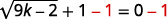Simplify.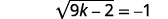Because the square root is equal to a negative number, the equation has no solution.

##### Exercise $$\PageIndex{3}$$

Solve: $$\sqrt{2 r-3}+5=0$$.

no solution

##### Exercise $$\PageIndex{4}$$

Solve: $$\sqrt{7 s-3}+2=0$$.

no solution

If one side of an equation with a square root is a binomial, we use the Product of Binomial Squares Pattern when we square it.

##### Definition: Binomial Squares

$$\begin{array}{l}{(a+b)^{2}=a^{2}+2 a b+b^{2}} \\ {(a-b)^{2}=a^{2}-2 a b+b^{2}}\end{array}$$

Don't forget the middle term!

##### Example $$\PageIndex{3}$$

Solve: $$\sqrt{p-1}+1=p$$.

Solution: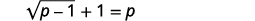To isolate the radical, subtract $$1$$ from both sides.Simplify.Square both sides of the equation.Simplify, using the Product of Binomial Squares Pattern on the right, Then solve the new equation.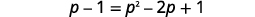It is a quadratic equation, so get zero on one side.Factor the right side.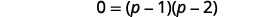Use the Zero Product Property.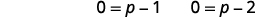Solve each equation.Check the answers.The solutions are $$p=1, p=2$$.

##### Exercise $$\PageIndex{5}$$

Solve: $$\sqrt{x-2}+2=x$$.

$$x=2, x=3$$

##### Exercise $$\PageIndex{6}$$

Solve: $$\sqrt{y-5}+5=y$$.

$$y=5, y=6$$

When the index of the radical is $$3$$, we cube both sides to remove the radical.

$$(\sqrt{a})^{3}=a$$

##### Example $$\PageIndex{4}$$

Solve: $$\sqrt{5 x+1}+8=4$$.

Solution:

 $$\sqrt{5 x+1}+8=4$$ To isolate the radical, subtract $$8$$ from both sides. $$\sqrt{5 x+1}=-4$$ Cube both sides of the equation. $$(\sqrt{5 x+1})^{3}=(-4)^{3}$$ Simplify. $$5 x+1=-64$$ Solve the equation. $$5 x=-65$$ $$x=-13$$ Check the answer.The solution is $$x=-13$$.
##### Exercise $$\PageIndex{7}$$

Solve: $$\sqrt{4 x-3}+8=5$$

$$x=-6$$

##### Exercise $$\PageIndex{8}$$

Solve: $$\sqrt{6 x-10}+1=-3$$

$$x=-9$$

Sometimes an equation will contain rational exponents instead of a radical. We use the same techniques to solve the equation as when we have a radical. We raise each side of the equation to the power of the denominator of the rational exponent. Since $$\left(a^{m}\right)^{^{n}}=a^{m \cdot n}$$, we have for example,

$$\left(x^{\frac{1}{2}}\right)^{2}=x,\left(x^{\frac{1}{3}}\right)^{3}=x$$

Remember, $$x^{\frac{1}{2}}=\sqrt{x}$$ and $$x^{\frac{1}{3}}=\sqrt{x}$$.

##### Example $$\PageIndex{5}$$

Solve: $$(3 x-2)^{\frac{1}{4}}+3=5$$.

Solution:

 $$(3 x-2)^{\frac{1}{4}}+3=5$$ To isolate the term with the rational exponent, subtract $$3$$ from both sides. $$(3 x-2)^{\frac{1}{4}}=2$$ Raise each side of the equation to the fourth power. $$\left((3 x-2)^{\frac{1}{4}}\right)^{4}=(2)^{4}$$ Simplify. $$3 x-2=16$$ Solve the equation. $$3x=18$$ $$x=6$$ Check the answer.The solution is $$x=6$$.
##### Exercise $$\PageIndex{9}$$

Solve: $$(9 x+9)^{\frac{1}{4}}-2=1$$

$$x=8$$

##### Exercise $$\PageIndex{10}$$

Solve: $$(4 x-8)^{\frac{1}{4}}+5=7$$

$$x=6$$

Sometimes the solution of a radical equation results in two algebraic solutions, but one of them may be an extraneous solution!

##### Example $$\PageIndex{6}$$

Solve: $$\sqrt{r+4}-r+2=0$$.

Solution:

 $$\sqrt{r+4}-r+2=0$$ Isolate the radical. $$\sqrt{r+4}=r-2$$ Square both sides of the equation. $$(\sqrt{r+4})^{2}=(r-2)^{2}$$ Simplify and then solve the equation. $$r+4=r^{2}-4 r+4$$ If it is a quadratic equation, so get zero on one side. $$0=r^{2}-5 r$$ Factor the right side. $$0=r(r-5)$$ Use the Zero Product Property. $$0=r \quad 0=r-5$$ Solve the equation. $$r=0 \quad r=5$$ Check your answer.The solution is $$r=5$$. $$r=0$$ is an extreneous solution.
##### Exercise $$\PageIndex{11}$$

Solve: $$\sqrt{m+9}-m+3=0$$

$$m=7$$

##### Exercise $$\PageIndex{12}$$

Solve: $$\sqrt{n+1}-n+1=0$$.

$$n=3$$

When there is a coefficient in front of the radical, we must raise it to the power of the index, too.

##### Example $$\PageIndex{7}$$

Solve: $$3 \sqrt{3 x-5}-8=4$$.

Solution:

 $$3 \sqrt{3 x-5}-8=4$$ Isolate the radical term. $$3 \sqrt{3 x-5}=12$$ Isolate the radical by dividing both sides by $$3$$. $$\sqrt{3 x-5}=4$$ Square both sides of the equation. $$(\sqrt{3 x-5})^{2}=(4)^{2}$$ Simplify, then solve the new equation. $$3 x-5=16$$ $$3x=21$$ Solve the equation. $$x=7$$ Check the answer.The solution is $$x=7$$.
##### Exercise $$\PageIndex{13}$$

Solve: $$2 \sqrt{4 a+4}-16=16$$.

$$a=63$$

##### Exercise $$\PageIndex{14}$$

Solve: $$3 \sqrt{2 b+3}-25=50$$

$$b=311$$

If the radical equation has two radicals, we start out by isolating one of them. It often works out easiest to isolate the more complicated radical first.

In the next example, when one radical is isolated, the second radical is also isolated.

##### Example $$\PageIndex{8}$$

Solve: $$\sqrt{4 x-3}=\sqrt{3 x+2}$$.

Solution:

$$\sqrt{4 x-3}=\sqrt{3 x+2}$$

Since the index is $$3$$, cube both sides of the equation.

$$(\sqrt{4 x-3})^{3}=(\sqrt{3 x+2})^{3}$$

Simplify, then solve the new equation.

\begin{aligned} 4 x-3 &=3 x+2 \\ x-3 &=2 \\ x &=5 \end{aligned}

The solution is $$x=5$$.

We leave it to you to show that $$5$$ checks!

##### Exercise $$\PageIndex{15}$$

Solve: $$\sqrt{5 x-4}=\sqrt{2 x+5}$$.

$$x=3$$

##### Exercise $$\PageIndex{16}$$

Solve: $$\sqrt{7 x+1}=\sqrt{2 x-5}$$.

$$x=-\frac{6}{5}$$

Sometimes after raising both sides of an equation to a power, we still have a variable inside a radical. When that happens, we repeat Step 1 and Step 2 of our procedure. We isolate the radical and raise both sides of the equation to the power of the index again.

##### Example $$\PageIndex{9}$$ how to solve a radical equation

Solve: $$\sqrt{m}+1=\sqrt{m+9}$$.

Solution:

 Step 1: Isolate one of the radical terms on one side of the equation. The radical on the right is isolated. $$\sqrt{m}+1=\sqrt{m+9}$$ Step 2: Raise both sides of the equation to the power of the index. We square both sides. Simplify--be very careful as you multiply! $$(\sqrt{m}+1)^{2}=(\sqrt{m+9})^{2}$$ Step 3: Are there any more radicals? If yes, repeat Step 1 and Step 2 again. If no, solve the new equation. There is still a radical in the equation. So we must repeat the previous steps. Isolate the radical term. Here, we can easily isolate the radical by dividing both sides by $$2$$. Square both sides. \begin{aligned} m+2 \sqrt{m}+1 &=m+9 \\ 2 \sqrt{m} &=8 \\ \sqrt{m} &=4 \\(\sqrt{m})^{2} &=(4)^{2} \\ m &=16 \end{aligned} Step 4: Check the answer in the original equation. \begin{aligned}\sqrt{m}+1&=\sqrt{m+9} \\ \sqrt{\color{red}{16}}\color{black}{+}1& \stackrel{?}{=} \sqrt{\color{red}{16}\color{black}{+}9} \\ 4+1& \stackrel{?}{=} 5 \\ 5&=5\end{aligned} The solution is $$m=16$$.
##### Exercise $$\PageIndex{17}$$

Solve: $$3-\sqrt{x}=\sqrt{x-3}$$.

$$x=4$$

##### Exercise $$\PageIndex{18}$$

Solve: $$\sqrt{x}+2=\sqrt{x+16}$$.

$$x=9$$

We summarize the steps here. We have adjusted our previous steps to include more than one radical in the equation This procedure will now work for any radical equations.

1. Isolate one of the radical terms on one side of the equation.
2. Raise both sides of the equation to the power of the index.
3. Are there any more radicals?
If yes, repeat Step 1 and Step 2 again.
If no, solve the new equation.
4. Check the answer in the original equation.

Be careful as you square binomials in the next example. Remember the pattern in $$(a+b)^{2}=a^{2}+2 a b+b^{2}$$ or $$(a-b)^{2}=a^{2}-2 a b+b^{2}$$.

##### Example $$\PageIndex{10}$$

Solve: $$\sqrt{q-2}+3=\sqrt{4 q+1}$$.

Solution:The radical on the right is isolated. Square both sides.Simplify.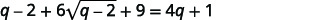There is still a radical in the equation so we must repeat the previous steps. Isolate the radical.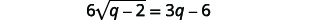Square both sides. It would not help to divide both sides by $$6$$. Remember to square both the $$6$$ and the $$\sqrt{q-2}$$.Simplify, then solve the new equation.Distribute.It is a quadratic equation, so get zero on one side.Factor the right side.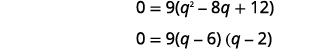Use the Zero Product Property.The checks are left to you. The solutions are $$q=6$$ and $$q=2$$.
##### Exercise $$\PageIndex{19}$$

Solve: $$\sqrt{x-1}+2=\sqrt{2 x+6}$$

$$x=5$$

##### Exercise $$\PageIndex{20}$$

Solve: $$\sqrt{x}+2=\sqrt{3 x+4}$$

$$x=0 x=4$$

As you progress through your college courses, you’ll encounter formulas that include radicals in many disciplines. We will modify our Problem Solving Strategy for Geometry Applications slightly to give us a plan for solving applications with formulas from any discipline.

### Use a Problem Solving Strategy for Applications With Formulas

1. Read the problem and make sure all the words and ideas are understood. When appropriate, draw a figure and label it with the given information.
2. Identify what we are looking for.
3. Name what we are looking for by choosing a variable to represent it.
4. Translate into an equation by writing the appropriate formula or model for the situation. Substitute in the given information.
5. Solve the equation using good algebra techniques.
6. Check the answer in the problem and make sure it makes sense.
7. Answer the question with a complete sentence.

One application of radicals has to do with the effect of gravity on falling objects. The formula allows us to determine how long it will take a fallen object to hit the gound.

##### Definition: Falling Objects

On Earth, if an object is dropped from a height of $$h$$ feet, the time in seconds it will take to reach the ground is found by using the formula

$$t=\frac{\sqrt{h}}{4}$$

For example, if an object is dropped from a height of $$64$$ feet, we can find the time it takes to reach the ground by substituting $$h=64$$ into the formula.Take the square root of $$64$$.Simplify the fraction.It would take $$2$$ seconds for an object dropped from a height of $$64$$ feet to reach the ground.

##### Example $$\PageIndex{11}$$

Marissa dropped her sunglasses from a bridge $$400$$ feet above a river. Use the formula $$t=\frac{\sqrt{h}}{4}$$ to find how many seconds it took for the sunglasses to reach the river.

Solution:

 Step 1: Read the problem. Step 2: Identify what we are looking. The time it takes for the sunglasses to reach the river. Step 3: Name what we are looking. Let (t=\) time. Step 4: Translate into an equation by writing the appropriate formula. Substitute in the given information.Step 5: Solve the equation.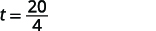Step 6: Check the answer in the problem and make sure it makes sense.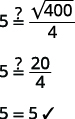Does $$5$$ seconds seem like a reasonable length of time? Yes. Step 7: Answer the equation. It will take $$5$$ seconds for the sunglasses to reach the river.
##### Exercise $$\PageIndex{21}$$

A helicopter dropped a rescue package from a height of $$1,296$$ feet. Use the formula $$t=\frac{\sqrt{h}}{4}$$ to find how many seconds it took for the package to reach the ground.

$$9$$ seconds

##### Exercise $$\PageIndex{22}$$

A window washer dropped a squeegee from a platform $$196$$ feet above the sidewalk. Use the formula $$t=\frac{\sqrt{h}}{4}$$ to find how many seconds it took for the squeegee to reach the sidewalk.

$$3.5$$ seconds

Police officers investigating car accidents measure the length of the skid marks on the pavement. Then they use square roots to determine the speed, in miles per hour, a car was going before applying the brakes.

##### Definition: Skid Marks and Speed of a Car

If the length of the skid marks is $$d$$ feet, then the speed, $$s$$, of the car before the brakes were applied can be found by using the formula

$$s=\sqrt{24 d}$$

##### Example $$\PageIndex{12}$$

After a car accident, the skid marks for one car measured $$190$$ feet. Use the formula $$s=\sqrt{24d}$$ to find the speed of the car before the brakes were applied. Round your answer to the nearest tenth.

Solution:

 Step 1: Read the problem. Step 2: Identify what we are looking for. The speed of a car. Step 3: Name what we are looking for. Let $$s=$$ the speed. Step 4: Translate into an equation by writing the appropriate formula. Substitute in the given information.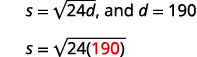Step 5: Solve the equation.Round to $$1$$ decimal place.The speed of the car before the brakes were applied was $$67.5$$ miles per hour.
##### Exercise $$\PageIndex{23}$$

An accident investigator measured the skid marks of the car. The length of the skid marks was $$76$$ feet. Use the formula $$s=\sqrt{24d}$$ to find the speed of the car before the brakes were applied. Round your answer to the nearest tenth.

$$42.7$$ mph

##### Exercise $$\PageIndex{24}$$

The skid marks of a vehicle involved in an accident were $$122$$ feet long. Use the formula $$s=\sqrt{24d}$$ to find the speed of the vehicle before the brakes were applied. Round your answer to the nearest tenth.

$$54.1$$ mph

Access these online resources for additional instruction and practice with solving radical equations.

• Solving an Equation Involving a Single Radical
• Solving Equations with Radicals and Rational Exponents

## Key Concepts

• Binomial Squares
$$\begin{array}{l}{(a+b)^{2}=a^{2}+2 a b+b^{2}} \\ {(a-b)^{2}=a^{2}-2 a b+b^{2}}\end{array}$$
1. Isolate one of the radical terms on one side of the equation.
2. Raise both sides of the equation to the power of the index.
3. Are there any more radicals?
If yes, repeat Step 1 and Step 2 again.
If no, solve the new equation.
4. Check the answer in the original equation.
• Problem Solving Strategy for Applications with Formulas
1. Read the problem and make sure all the words and ideas are understood. When appropriate, draw a figure and label it with the given information.
2. Identify what we are looking for.
3. Name what we are looking for by choosing a variable to represent it.
4. Translate into an equation by writing the appropriate formula or model for the situation. Substitute in the given information.
5. Solve the equation using good algebra techniques.
6. Check the answer in the problem and make sure it makes sense.
7. Answer the question with a complete sentence.
• Falling Objects
• On Earth, if an object is dropped from a height of $$h$$ feet, the time in seconds it will take to reach the ground is found by using the formula $$t=\frac{\sqrt{h}}{4}$$.
• Skid Marks and Speed of a Car
• If the length of the skid marks is $$d$$ feet, then the speed, $$s$$, of the car before the brakes were applied can be found by using the formula $$s=\sqrt{24d}$$.